Test: Single Phase FW AC-DC - 1

# Test: Single Phase FW AC-DC - 1

Test Description

## 30 Questions MCQ Test Power Electronics | Test: Single Phase FW AC-DC - 1

Test: Single Phase FW AC-DC - 1 for Electrical Engineering (EE) 2023 is part of Power Electronics preparation. The Test: Single Phase FW AC-DC - 1 questions and answers have been prepared according to the Electrical Engineering (EE) exam syllabus.The Test: Single Phase FW AC-DC - 1 MCQs are made for Electrical Engineering (EE) 2023 Exam. Find important definitions, questions, notes, meanings, examples, exercises, MCQs and online tests for Test: Single Phase FW AC-DC - 1 below.
Solutions of Test: Single Phase FW AC-DC - 1 questions in English are available as part of our Power Electronics for Electrical Engineering (EE) & Test: Single Phase FW AC-DC - 1 solutions in Hindi for Power Electronics course. Download more important topics, notes, lectures and mock test series for Electrical Engineering (EE) Exam by signing up for free. Attempt Test: Single Phase FW AC-DC - 1 | 30 questions in 25 minutes | Mock test for Electrical Engineering (EE) preparation | Free important questions MCQ to study Power Electronics for Electrical Engineering (EE) Exam | Download free PDF with solutions
 1 Crore+ students have signed up on EduRev. Have you?
Test: Single Phase FW AC-DC - 1 - Question 1

### The below shown single phase controlled rectifier configuration is a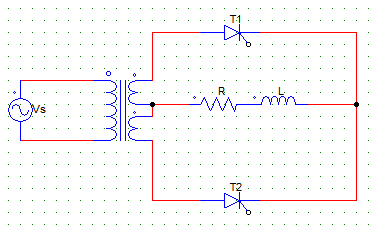Detailed Solution for Test: Single Phase FW AC-DC - 1 - Question 1

It is a two pulse i.e full wave M-2 connection.

Test: Single Phase FW AC-DC - 1 - Question 2

### In the below shown circuit, considering the load to be purely R load, the thyristor T2 would conduct from ωt =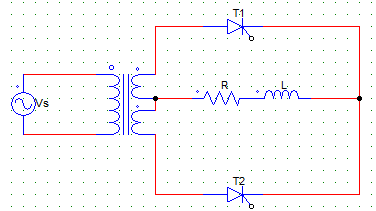Detailed Solution for Test: Single Phase FW AC-DC - 1 - Question 2

The bottom winding forward biases the SCR T2 at π, conduction starts after the pulse is given at α & stops when T2 is naturally commutated at 2π.

Test: Single Phase FW AC-DC - 1 - Question 3

### For a single pulse M-2 rectifier with RL load and firing angle α >0°, the value of the inductance L is such that the load current is continuous.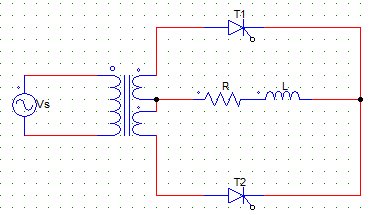Choose the correct statement.

Detailed Solution for Test: Single Phase FW AC-DC - 1 - Question 3

As mentioned, L is such that the load current is continuous. Hence, it never falls below a predefined positive value.

Test: Single Phase FW AC-DC - 1 - Question 4

For the circuit below, the transformer ratio is 1:1 and Vs = Vm sinωt. When T1 is forward biased and is triggered at α than T2 is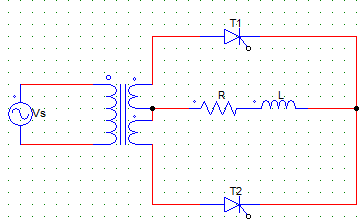Detailed Solution for Test: Single Phase FW AC-DC - 1 - Question 4

When T1 is gated, the other SCR T2 is reversed biased due to the windings so connected.

Test: Single Phase FW AC-DC - 1 - Question 5

The circuit turn-off time of M-2 type connection is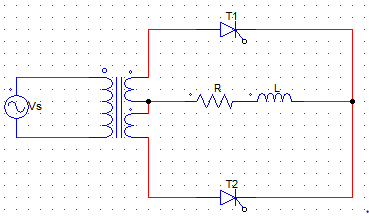Detailed Solution for Test: Single Phase FW AC-DC - 1 - Question 5

When T1 is gated, SCR T2 is off from ωt = α to π
Thus t = π-α(offtime)/ω
Same can be said when T2 is gated and T2 is off.

Test: Single Phase FW AC-DC - 1 - Question 6

Find the expression for the average value of the output voltage for the below given circuit. Consider the load current to be continuous, firing angle = α, transformer ration 1:1 and Vs = Vm sinωt.

Detailed Solution for Test: Single Phase FW AC-DC - 1 - Question 6

As the load current is continues, the voltage is positive from α to π and negative from π to π+α and so on. Thus,
Vavg = 1/Period ∫ Vm sin⁡ωt d(ωt)
Where period = π
And the integral runs from α to α+π.

Test: Single Phase FW AC-DC - 1 - Question 7

A fully controlled converter uses

Detailed Solution for Test: Single Phase FW AC-DC - 1 - Question 7

Fully controlled implys that all the elements are “fully controlled” hence, it uses SCRs only except the FD.

Test: Single Phase FW AC-DC - 1 - Question 8

A single phase full-converter using R load is a _________ quadrant converter and that using an RL load without FD is a __________ quadrant converter

Detailed Solution for Test: Single Phase FW AC-DC - 1 - Question 8

In R load both V and I are positive, in RL load the voltage can be negative but current is always positive.

Test: Single Phase FW AC-DC - 1 - Question 9

A single phase full controlled bridge converter (B-2) uses

Detailed Solution for Test: Single Phase FW AC-DC - 1 - Question 9

4 SCR’s are connected in a bridge fashion.

Test: Single Phase FW AC-DC - 1 - Question 10

In a B-2 type full controlled bridge converter

Detailed Solution for Test: Single Phase FW AC-DC - 1 - Question 10

B-2 is the bridge type controller, in which 2 devices conduct at a time. One acting as the current supplying path and other acts as a return path.

Test: Single Phase FW AC-DC - 1 - Question 11

For the below given circuit,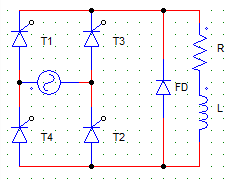Detailed Solution for Test: Single Phase FW AC-DC - 1 - Question 11

T1-T2 are gated together at a time and T3-T4 are gated together at a time.

Test: Single Phase FW AC-DC - 1 - Question 12

With respect to the below given circuit, from ωt = 0 to α, ____ thyristor(s) block the forward voltage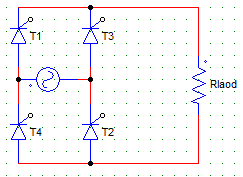Detailed Solution for Test: Single Phase FW AC-DC - 1 - Question 12

T1 and T2 are not yet gated, hence they block the forward voltage.

Test: Single Phase FW AC-DC - 1 - Question 13

With respect to the below given circuit, from ωt = 0 to α,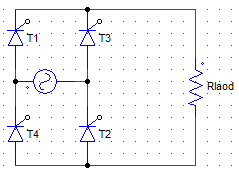Detailed Solution for Test: Single Phase FW AC-DC - 1 - Question 13

T1 and T2 are not yet gated, hence they block the forward voltage where as no voltage exists across T3 and T4.

Test: Single Phase FW AC-DC - 1 - Question 14

For the below given circuit, when the devices T1 and T2 are conducting from ωt = α till they are naturally commutated at π voltage across the other two pair of devices is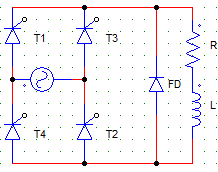Detailed Solution for Test: Single Phase FW AC-DC - 1 - Question 14

When T1 and T2 are conducting they act like S.C’s and the other pair of devices i.e. T3 and T4 has to take care of the reverse voltage.

Test: Single Phase FW AC-DC - 1 - Question 15

A single-phase full converter B-2 type connection has a RLE type of motor load connected. The minimum requirement to turn-on the device is

Detailed Solution for Test: Single Phase FW AC-DC - 1 - Question 15

Device will not turn on until the supply voltage exceeds the motor back emf E.

Test: Single Phase FW AC-DC - 1 - Question 16

For the below shown circuit with continuous load current.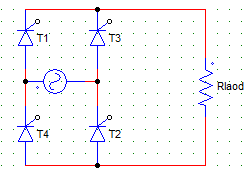Each thyristor pair conducts for

Detailed Solution for Test: Single Phase FW AC-DC - 1 - Question 16

As the load current is continuous each pair conducts from π to π+α i.e π radians.

Test: Single Phase FW AC-DC - 1 - Question 17

For the below given circuit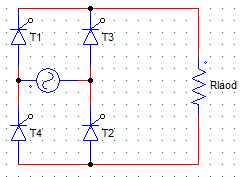Detailed Solution for Test: Single Phase FW AC-DC - 1 - Question 17

T1-T2 are gated together at a time and T3-T4 are gated together at a time.

Test: Single Phase FW AC-DC - 1 - Question 18

For the below shown circuit has dis-continuous load current waveform.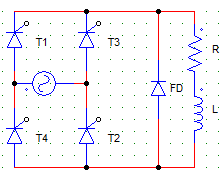Each thyristor pair conducts for

Detailed Solution for Test: Single Phase FW AC-DC - 1 - Question 18

Load current is discontinues than SCR pair conducts for less than 90° depending on the value of the inductor L.

Test: Single Phase FW AC-DC - 1 - Question 19

A single-phase full converter with B-2 type of connection has a continuous load current waveform. The thyristor pairs T3, T4 is triggered at ωt =

Detailed Solution for Test: Single Phase FW AC-DC - 1 - Question 19

First, at α T1-T2 conduct, then after π+α the next pair is excited i.e. T3-T4 and likewise.

Test: Single Phase FW AC-DC - 1 - Question 20

The PIV for each diode in a single-phase, full converter with B-2 type of controlled rectifier is

Consider supply voltage as Vs = Vm sinωt.

Detailed Solution for Test: Single Phase FW AC-DC - 1 - Question 20

The peak inverse voltage handled by each device is Vm in case of B-2 type of configuration.

Test: Single Phase FW AC-DC - 1 - Question 21

A motor load is connected to a single-phase full converter B-2 type controlled rectifier. The net energy is transferred from ac source to the motor (dc load) when

Detailed Solution for Test: Single Phase FW AC-DC - 1 - Question 21

Converter will work as a line commuted inverter when π-α > α.

Test: Single Phase FW AC-DC - 1 - Question 22

The below shown rectifier configuration has continues load current, find the expression for the average value of output voltage when the supply Vs = Vm sinωt is connected.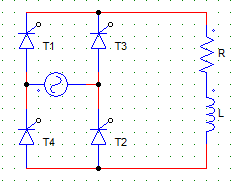Detailed Solution for Test: Single Phase FW AC-DC - 1 - Question 22

Vo = 1/π x [∫ Vm sinωt d(ωt)] Where the integration runs from α to π+α.

Test: Single Phase FW AC-DC - 1 - Question 23

Find the expression of the rms value of output voltage for a single-phase M-2 type rectifier with RL load and continues load current. Transformer ratio is 1:1 with supply voltage Vs = Vm sinωt

Detailed Solution for Test: Single Phase FW AC-DC - 1 - Question 23

Vor2 = 1/π x [∫ Vm2 sin2ωt d(ωt)], Where the integration runs from α to π+α
Vor = Vm2/2 = Vs.

Test: Single Phase FW AC-DC - 1 - Question 24

A single-phase full controlled converted with RLE load will act like a line-commutated inverter when the firing angle α

Detailed Solution for Test: Single Phase FW AC-DC - 1 - Question 24

It will act like a inverter i.e. most of the current would flow from the battery or back emf E to the source. For α=90° it will not act like a converter nor an inverter.

Test: Single Phase FW AC-DC - 1 - Question 25

In converter operation, with output voltage = Vo and RLE load.

Detailed Solution for Test: Single Phase FW AC-DC - 1 - Question 25

The output voltage obtained by a converter is always greater than the counter or back emf E.
Vo = IR + E.

Test: Single Phase FW AC-DC - 1 - Question 26

In inverter operation, with output voltage = Vo and a RLE load connected

Detailed Solution for Test: Single Phase FW AC-DC - 1 - Question 26

In inverter operation Vo < E.

Test: Single Phase FW AC-DC - 1 - Question 27

A motor load is connected to a single-phase full converter B-2 type controlled rectifier, the net energy is transferred from ac source to the motor (dc load) when

Detailed Solution for Test: Single Phase FW AC-DC - 1 - Question 27

It works as a converter when π-α < α.

Test: Single Phase FW AC-DC - 1 - Question 28

The below shown rectifier configuration has continues load current, find the expression of the RMS value of output voltage when the supply Vs = Vm sinωt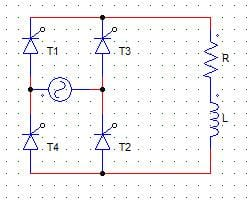Detailed Solution for Test: Single Phase FW AC-DC - 1 - Question 28

Vor2 = 1/π x [∫Vm2 sin2ωt d(ωt)] Where the integration runs from α to π+α
Vor = Vm2/2 = Vs.

Test: Single Phase FW AC-DC - 1 - Question 29

Choose the correct statement

Detailed Solution for Test: Single Phase FW AC-DC - 1 - Question 29

The PIV of diodes in M-2 is 2Vm, whereas that in B-2 type of connection is Vm.

Test: Single Phase FW AC-DC - 1 - Question 30

An SCR has the peak forward voltage = 1000 V. Find the maximum voltage that the SCR can handle if employed in a M-2 type full controlled converter circuit. Use factor of safety (FOS) = 2.5

Detailed Solution for Test: Single Phase FW AC-DC - 1 - Question 30

In M-2 type configuration maximum voltage handled is 2Vm
Therefore, 1000/2×2.5 = 200 V.

## Power Electronics

5 videos|39 docs|63 tests
 Use Code STAYHOME200 and get INR 200 additional OFF Use Coupon Code
Information about Test: Single Phase FW AC-DC - 1 Page
In this test you can find the Exam questions for Test: Single Phase FW AC-DC - 1 solved & explained in the simplest way possible. Besides giving Questions and answers for Test: Single Phase FW AC-DC - 1, EduRev gives you an ample number of Online tests for practice

## Power Electronics

5 videos|39 docs|63 tests